# SSAT Upper Level Math : How to find transformation for an analytic geometry equation

## Example Questions

2 Next →

### Example Question #471 : Ssat Upper Level Quantitative (Math)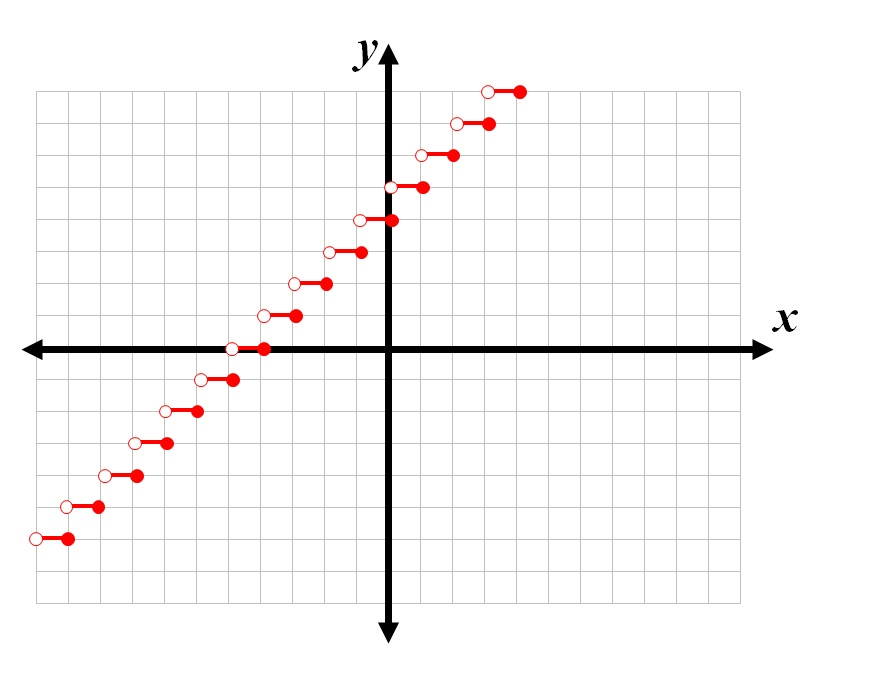What equation is graphed in the above figure?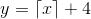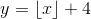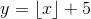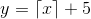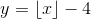Explanation:

The least integer function, or celing function,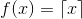, pairs each value ofwith the least integer greater than or equal to. Its graph is below.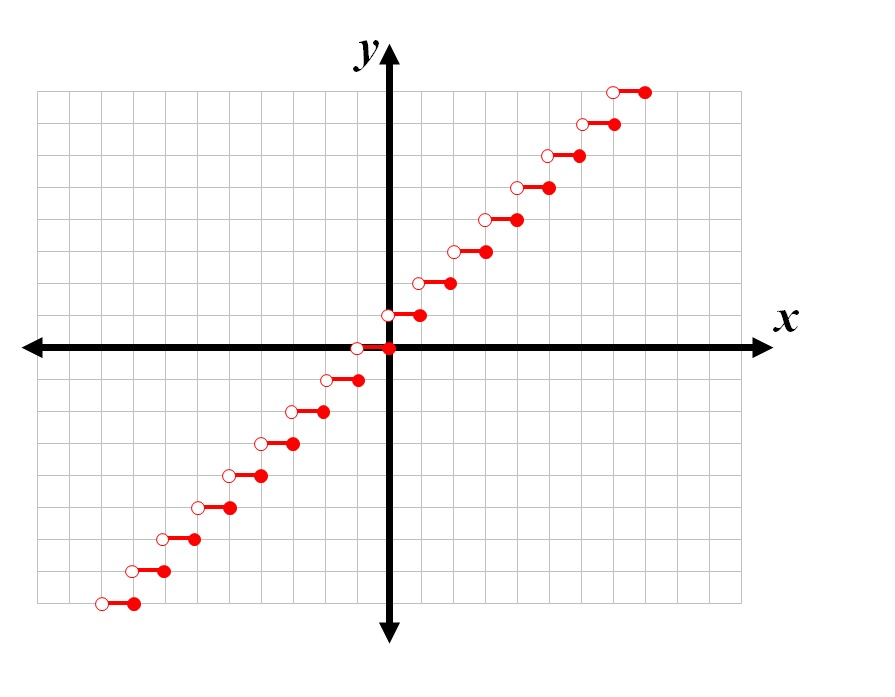The given graph is the above graph shifted upward four units. The graph of any function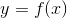shifted upward four units is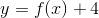, so the given graph corresponds to equation.

### Example Question #11 : How To Find Transformation For An Analytic Geometry Equation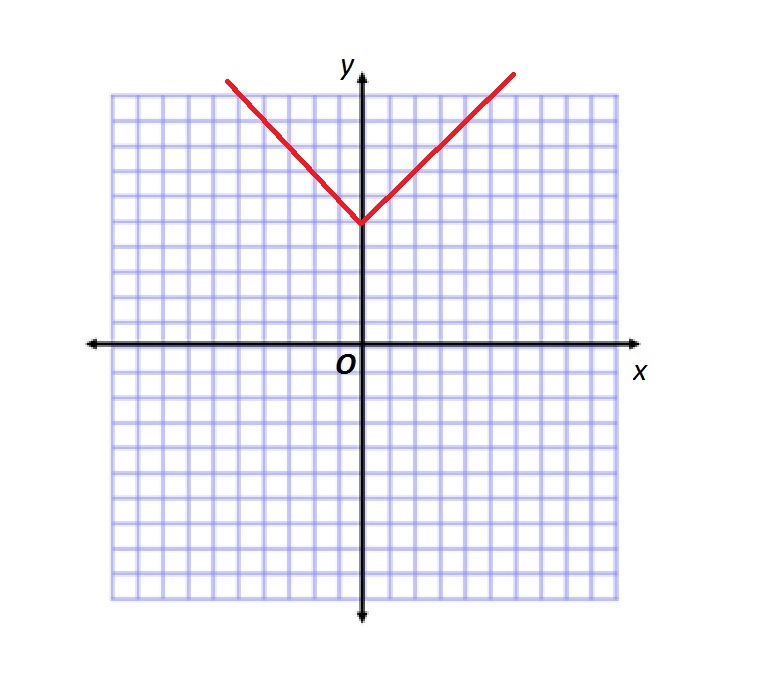Give the equation graphed in the above figure.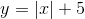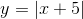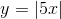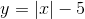The graph below is the graph of the absolute value function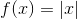, which pairs each-coordinate with its absolute value.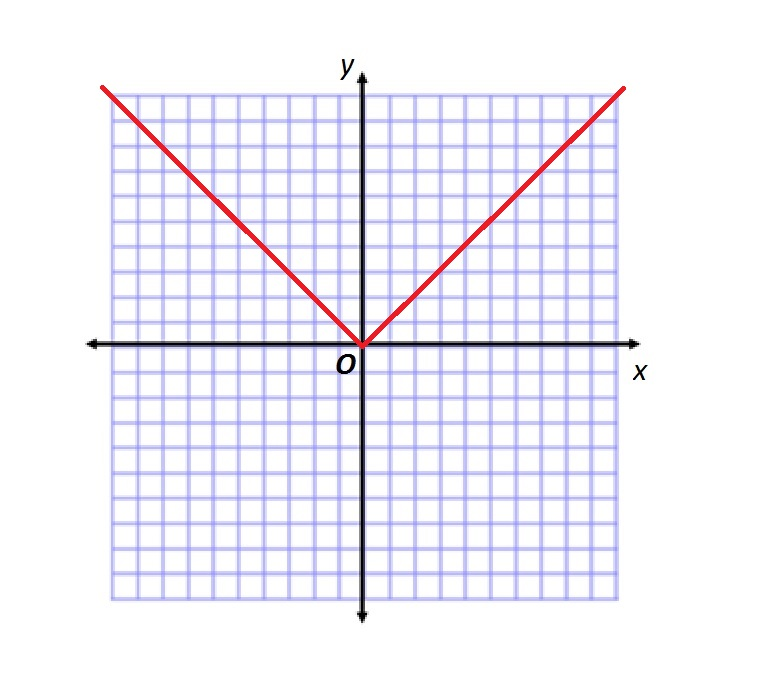The given graph is the above graph shifted upward five units. The graph of any functionshifted upward five units is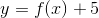, so the correct response is.